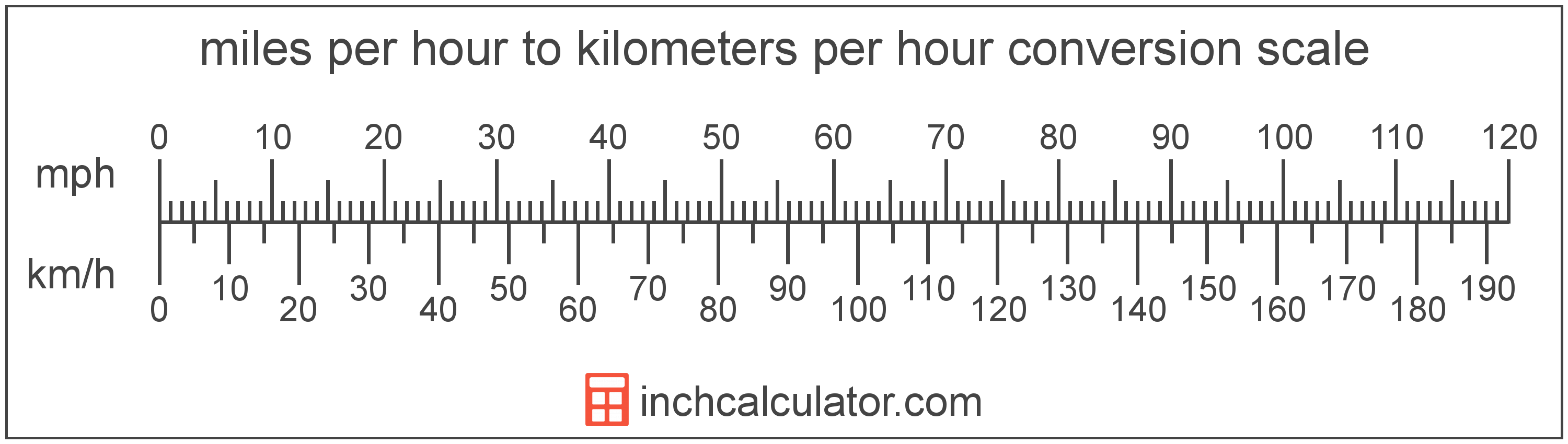# Kilometers per Hour to Miles per Hour Converter

Enter the speed in kilometers per hour below to get the value converted to miles per hour.

Results in Miles per Hour:1 km/h = 0.621371 mph

Do you want to convert miles per hour to kilometers per hour?

## How to Convert Kilometers per Hour to Miles per Hour

The fastest way to convert kilometers per hour to miles per hour is to use this simple formula:

mph = km/h ÷ 1.609344

The speed in miles per hour is equal to the speed in kilometers per hour divided by 1.609344. Since one kilometer per hour is equal to 1.609344 miles per hour, that's the conversion ratio used in the formula.

For example, here's how to convert 5 kilometers per hour to miles per hour using the formula above.

mph = (5 km/h ÷ 1.609344) = 3.106856 mphKilometers per hour and miles per hour are both units used to measure speed. Calculate speed in kilometers per hour or miles per hour using our speed calculator or keep reading to learn more about each unit of measure.

## What Are Kilometers per Hour?

Kilometers per hour are a measurement of speed expressing the distance traveled in kilometers in one hour.

The kilometer per hour, or kilometre per hour, is an SI unit of speed in the metric system. Kilometers per hour can be abbreviated as km/h, and are also sometimes abbreviated as kph. For example, 1 kilometer per hour can be written as 1 km/h or 1 kph.

In the expressions of units, the slash, or solidus (/), is used to express a change in one or more units relative to a change in one or more other units. For example, km/h is expressing a change in length or distance relative to a change in time.

Kilometers per hour can be expressed using the formula:
vkm/h = dkm / thr

The velocity in kilometers per hour is equal to the distance in kilometers divided by time in hours.

## What Are Miles per Hour?

Miles per hour are a measurement of speed expressing the distance traveled in miles in one hour.

The mile per hour is a US customary and imperial unit of speed. Miles per hour can be abbreviated as mph, and are also sometimes abbreviated as mi/h or MPH. For example, 1 mile per hour can be written as 1 mph, 1 mi/h, or 1 MPH.

Miles per hour can be expressed using the formula:
vmph = dmi / thr

The velocity in miles per hour is equal to the distance in miles divided by time in hours.

## Kilometer per Hour to Mile per Hour Conversion Table

Kilometer per hour measurements converted to miles per hour
Kilometers Per Hour Miles Per Hour
1 km/h 0.62 mph
2 km/h 1.24 mph
3 km/h 1.86 mph
4 km/h 2.49 mph
5 km/h 3.11 mph
10 km/h 6.21 mph
15 km/h 9.32 mph
20 km/h 12.43 mph
25 km/h 15.53 mph
30 km/h 18.64 mph
35 km/h 21.75 mph
40 km/h 24.85 mph
45 km/h 27.96 mph
50 km/h 31.07 mph
55 km/h 34.18 mph
60 km/h 37.28 mph
65 km/h 40.39 mph
70 km/h 43.5 mph
75 km/h 46.6 mph
80 km/h 49.71 mph
85 km/h 52.82 mph
90 km/h 55.92 mph
95 km/h 59.03 mph
100 km/h 62.14 mph
105 km/h 65.24 mph
110 km/h 68.35 mph
115 km/h 71.46 mph
120 km/h 74.56 mph
125 km/h 77.67 mph
130 km/h 80.78 mph
135 km/h 83.89 mph
140 km/h 86.99 mph
145 km/h 90.1 mph
150 km/h 93.21 mph
155 km/h 96.31 mph
160 km/h 99.42 mph

## References

1. National Institute of Standards and Technology, NIST Guide to the SI, https://www.nist.gov/pml/special-publication-811/nist-guide-si-appendix-b-conversion-factors/nist-guide-si-appendix-b8
2. National Institute of Standards and Technology, NIST Guide to the SI, Chapter 6: Rules and Style Conventions for Printing and Using Units, https://www.nist.gov/pml/special-publication-811/nist-guide-si-chapter-6-rules-and-style-conventions-printing-and-using
3. Wikipedia, Miles per hour, https://en.wikipedia.org/wiki/Miles_per_hour